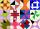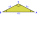# Isosceles triangle calculator (S) - result

Please enter two properties of the isosceles triangle

Use symbols: a,b c, h, T, p, A, B, C, r, R

You have entered area S and angle γ.

### Acute isosceles triangle.

Sides: a = 16.81879283051   b = 16.81879283051   c = 12.87218850581

Area: T = 100
Perimeter: p = 46.50877416683
Semiperimeter: s = 23.25438708341

Angle ∠ A = α = 67.5° = 67°30' = 1.17880972451 rad
Angle ∠ B = β = 67.5° = 67°30' = 1.17880972451 rad
Angle ∠ C = γ = 45° = 0.78553981634 rad

Height: ha = 11.892207115
Height: hb = 11.892207115
Height: hc = 15.53877397403

Median: ma = 12.3921666175
Median: mb = 12.3921666175
Median: mc = 15.53877397403

Vertex coordinates: A[12.87218850581; 0] B[0; 0] C[6.43659425291; 15.53877397403]
Centroid: CG[6.43659425291; 5.17992465801]
Coordinates of the circumscribed circle: U[6.43659425291; 6.43659425291]
Coordinates of the inscribed circle: I[6.43659425291; 4.33003593128]

Exterior (or external, outer) angles of the triangle:
∠ A' = α' = 112.5° = 112°30' = 1.17880972451 rad
∠ B' = β' = 112.5° = 112°30' = 1.17880972451 rad
∠ C' = γ' = 135° = 0.78553981634 rad

# How did we calculate this triangle?

The calculation of the triangle progress in two phases. The first phase is such that we try to calculate all three sides of the triangle from the input parameters. The first phase is different for the different triangles query entered. The second phase is the calculation of other characteristics of the triangle, such as angles, area, perimeter, heights, the center of gravity, circle radii, etc. Some input data also results in two to three correct triangle solutions (e.g., if the specified triangle area and two sides - typically resulting in both acute and obtuse) triangle).

### 3. From side a we calculate side b:

$b=a=16.818$

### 4. From side a we calculate perimeter p:

Now we know the lengths of all three sides of the triangle, and the triangle is uniquely determined. Next, we calculate another its characteristics - same procedure as calculation of the triangle from the known three sides SSS.

### 6. Semiperimeter of the triangle

The semiperimeter of the triangle is half its perimeter. The semiperimeter frequently appears in formulas for triangles that it is given a separate name. By the triangle inequality, the longest side length of a triangle is less than the semiperimeter.

### 7. The triangle area using Heron's formula

Heron's formula gives the area of a triangle when the length of all three sides are known. There is no need to calculate angles or other distances in the triangle first. Heron's formula works equally well in all cases and types of triangles.

### 8. Calculate the heights of the triangle from its area.

There are many ways to find the height of the triangle. The easiest way is from the area and base length. The area of a triangle is half of the product of the length of the base and the height. Every side of the triangle can be a base; there are three bases and three heights (altitudes). Triangle height is the perpendicular line segment from a vertex to a line containing the base.

### 9. Calculation of the inner angles of the triangle using a Law of Cosines

The Law of Cosines is useful for finding the angles of a triangle when we know all three sides. The cosine rule, also known as the law of cosines, relates all three sides of a triangle with an angle of a triangle. The Law of Cosines is the extrapolation of the Pythagorean theorem for any triangle. Pythagorean theorem works only in a right triangle. Pythagorean theorem is a special case of the Law of Cosines and can be derived from it because the cosine of 90° is 0. It is best to find the angle opposite the longest side first. With the Law of Cosines, there is also no problem with obtuse angles as with the Law of Sines, because cosine function is negative for obtuse angles, zero for right, and positive for acute angles. We also use inverse cosine called arccosine to determine the angle from cosine value.

An incircle of a triangle is a circle which is tangent to each side. An incircle center is called incenter and has a radius named inradius. All triangles have an incenter, and it always lies inside the triangle. The incenter is the intersection of the three angle bisectors. The product of the inradius and semiperimeter (half the perimeter) of a triangle is its area.

The circumcircle of a triangle is a circle that passes through all of the triangle's vertices, and the circumradius of a triangle is the radius of the triangle's circumcircle. Circumcenter (center of circumcircle) is the point where the perpendicular bisectors of a triangle intersect.

### 12. Calculation of medians

A median of a triangle is a line segment joining a vertex to the midpoint of the opposite side. Every triangle has three medians, and they all intersect each other at the triangle's centroid. The centroid divides each median into parts in the ratio 2:1, with the centroid being twice as close to the midpoint of a side as it is to the opposite vertex. We use Apollonius's theorem to calculate the length of a median from the lengths of its side.

### An isosceles triangle in word problems in mathematics:

• Isosceles triangleWhat are the angles of an isosceles triangle ABC if its base is long a=5 m and has an arm b=4 m.
• Isosceles - isoscelesIt is given a triangle ABC with sides /AB/ = 3 cm /BC/ = 10 cm, and the angle ABC = 120°. Draw all points X such that true that BCX triangle is an isosceles and triangle ABX is isosceles with the base AB.
• Isosceles IVIn an isosceles triangle ABC is |AC| = |BC| = 13 and |AB| = 10. Calculate the radius of the inscribed (r) and described (R) circle.
• Isosceles triangleThe circumference of the isosceles triangle is 32.5 dm. Base length is 153 cm. How long is the leg of this triangle?
• Isosceles right triangleArea of an isosceles right triangle is 18 dm2. Calculate the length of its base.
• Isosceles triangleThe leg of the isosceles triangle is 5 dm, its height is 20 cm longer than the base. Calculate base length z.
• Isosceles triangle 10In an isosceles triangle, the equal sides are 2/3 of the length of the base. Determine the measure of the base angles.
• Isosceles triangle 8If the rate of the sides an isosceles triangle is 7:6:7, find the base angle correct to the nearest degree.
• Isosceles triangleCalculate the area of an isosceles triangle, the base of which measures 16 cm and the arms 10 cm.
• Isosceles triangleCalculate the perimeter of isosceles triangle with arm length 73 cm and base length of 48 cm.
• Right isosceles triangleWhat can be the area of a right isosceles triangle with a side length of 8 cm?
• Isosceles triangleIn an isosceles triangle ABC with base AB; A [3,4]; B [1,6] and the vertex C lies on the line 5x - 6y - 16 = 0. Calculate the coordinates of vertex C.
• Medians of isosceles triangleThe isosceles triangle has a base ABC |AB| = 16 cm and 10 cm long arm. What are the length of medians?
• Isosceles right triangleCalculate the area of an isosceles right triangle whose perimeter is 252 cm.
• Right isosceles triangleRight isosceles triangle has an altitude x drawn from the right angle to the hypotenuse dividing it into 2 equal segments. The length of one segment is 5 cm. What is the area of the triangle?